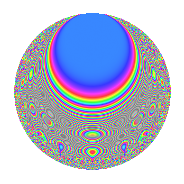Properties

 Label 4012.2.bLevel 4012 Weight 2 Character orbit b Rep. character $$\chi_{4012}(237,\cdot)$$ Character field $$\Q$$ Dimension 86 Newforms 2 Sturm bound 1080 Trace bound 1

Related objects

Defining parameters

 Level: $$N$$ = $$4012 = 2^{2} \cdot 17 \cdot 59$$ Weight: $$k$$ = $$2$$ Character orbit: $$[\chi]$$ = 4012.b (of order $$2$$ and degree $$1$$) Character conductor: $$\operatorname{cond}(\chi)$$ = $$17$$ Character field: $$\Q$$ Newforms: $$2$$ Sturm bound: $$1080$$ Trace bound: $$1$$

Dimensions

The following table gives the dimensions of various subspaces of $$M_{2}(4012, [\chi])$$.

Total New Old
Modular forms 546 86 460
Cusp forms 534 86 448
Eisenstein series 12 0 12

Trace form

 $$86q$$ $$\mathstrut -\mathstrut 90q^{9}$$ $$\mathstrut +\mathstrut O(q^{10})$$ $$86q$$ $$\mathstrut -\mathstrut 90q^{9}$$ $$\mathstrut +\mathstrut 16q^{13}$$ $$\mathstrut +\mathstrut 10q^{15}$$ $$\mathstrut -\mathstrut 7q^{17}$$ $$\mathstrut -\mathstrut 18q^{21}$$ $$\mathstrut -\mathstrut 78q^{25}$$ $$\mathstrut -\mathstrut 12q^{33}$$ $$\mathstrut -\mathstrut 26q^{35}$$ $$\mathstrut -\mathstrut 16q^{43}$$ $$\mathstrut -\mathstrut 4q^{47}$$ $$\mathstrut -\mathstrut 58q^{49}$$ $$\mathstrut +\mathstrut 20q^{51}$$ $$\mathstrut +\mathstrut 16q^{53}$$ $$\mathstrut -\mathstrut 32q^{55}$$ $$\mathstrut +\mathstrut 6q^{59}$$ $$\mathstrut +\mathstrut 24q^{67}$$ $$\mathstrut -\mathstrut 8q^{69}$$ $$\mathstrut -\mathstrut 16q^{77}$$ $$\mathstrut +\mathstrut 86q^{81}$$ $$\mathstrut -\mathstrut 20q^{83}$$ $$\mathstrut -\mathstrut 24q^{85}$$ $$\mathstrut +\mathstrut 26q^{87}$$ $$\mathstrut -\mathstrut 36q^{89}$$ $$\mathstrut -\mathstrut 4q^{93}$$ $$\mathstrut +\mathstrut O(q^{100})$$

Decomposition of $$S_{2}^{\mathrm{new}}(4012, [\chi])$$ into irreducible Hecke orbits

Label Dim. $$A$$ Field CM Traces $q$-expansion
$$a_2$$ $$a_3$$ $$a_5$$ $$a_7$$
4012.2.b.a $$40$$ $$32.036$$ None $$0$$ $$0$$ $$0$$ $$0$$
4012.2.b.b $$46$$ $$32.036$$ None $$0$$ $$0$$ $$0$$ $$0$$

Decomposition of $$S_{2}^{\mathrm{old}}(4012, [\chi])$$ into lower level spaces

$$S_{2}^{\mathrm{old}}(4012, [\chi]) \cong$$ $$S_{2}^{\mathrm{new}}(34, [\chi])$$$$^{\oplus 4}$$$$\oplus$$$$S_{2}^{\mathrm{new}}(68, [\chi])$$$$^{\oplus 2}$$$$\oplus$$$$S_{2}^{\mathrm{new}}(1003, [\chi])$$$$^{\oplus 3}$$$$\oplus$$$$S_{2}^{\mathrm{new}}(2006, [\chi])$$$$^{\oplus 2}$$